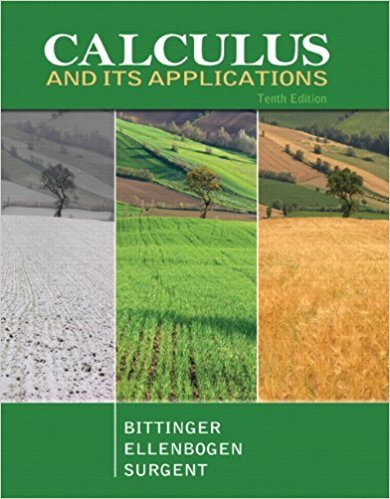×
×

# Solutions for Chapter 1.8: Higher-Order Derivatives## Full solutions for Calculus and Its Applications | 10th Edition

ISBN: 9780321694331Solutions for Chapter 1.8: Higher-Order Derivatives

Solutions for Chapter 1.8
4 5 0 295 Reviews
16
3
##### ISBN: 9780321694331

Chapter 1.8: Higher-Order Derivatives includes 77 full step-by-step solutions. This expansive textbook survival guide covers the following chapters and their solutions. This textbook survival guide was created for the textbook: Calculus and Its Applications, edition: 10. Since 77 problems in chapter 1.8: Higher-Order Derivatives have been answered, more than 24253 students have viewed full step-by-step solutions from this chapter. Calculus and Its Applications was written by and is associated to the ISBN: 9780321694331.

Key Calculus Terms and definitions covered in this textbook
• Angle

Union of two rays with a common endpoint (the vertex). The beginning ray (the initial side) can be rotated about its endpoint to obtain the final position (the terminal side)

• Dependent event

An event whose probability depends on another event already occurring

• Directed angle

See Polar coordinates.

• Equivalent vectors

Vectors with the same magnitude and direction.

• Event

A subset of a sample space.

• Half-life

The amount of time required for half of a radioactive substance to decay.

• Infinite limit

A special case of a limit that does not exist.

• Intermediate Value Theorem

If ƒ is a polynomial function and a < b , then ƒ assumes every value between ƒ(a) and ƒ(b).

• Interquartile range

The difference between the third quartile and the first quartile.

• Limit to growth

See Logistic growth function.

• Logarithmic form

An equation written with logarithms instead of exponents

• Mapping

A function viewed as a mapping of the elements of the domain onto the elements of the range

• Mean (of a set of data)

The sum of all the data divided by the total number of items

• Nautical mile

Length of 1 minute of arc along the Earth’s equator.

• One-to-one rule of logarithms

x = y if and only if logb x = logb y.

• Random numbers

Numbers that can be used by researchers to simulate randomness in scientific studies (they are usually obtained from lengthy tables of decimal digits that have been generated by verifiably random natural phenomena).

• Rational expression

An expression that can be written as a ratio of two polynomials.

• Spiral of Archimedes

The graph of the polar curve.

• Triangular form

A special form for a system of linear equations that facilitates finding the solution.

• xy-plane

The points x, y, 0 in Cartesian space.

×